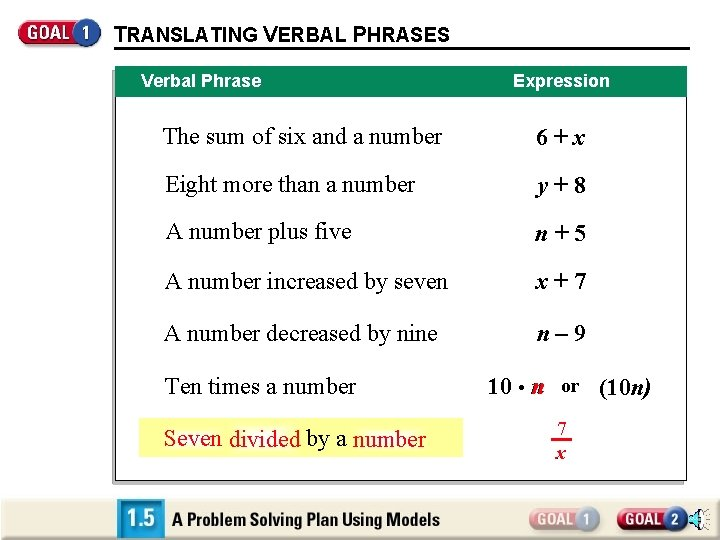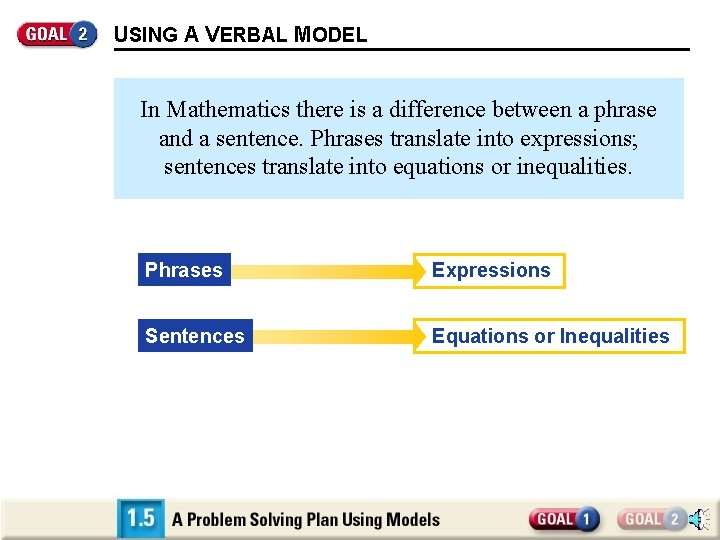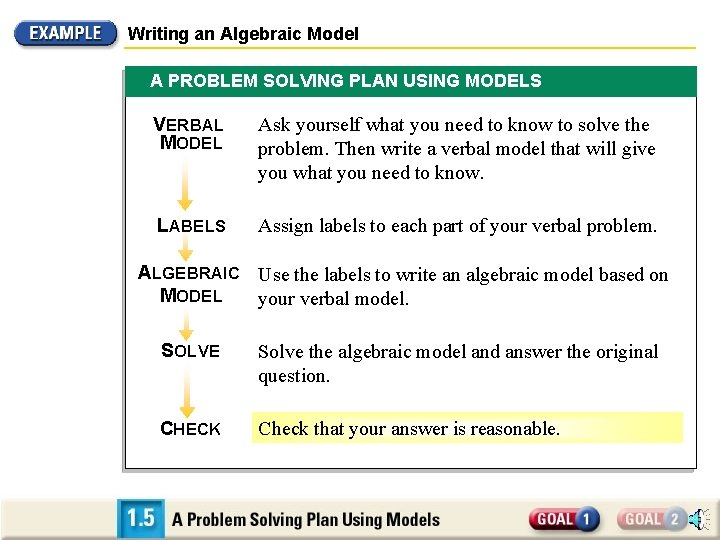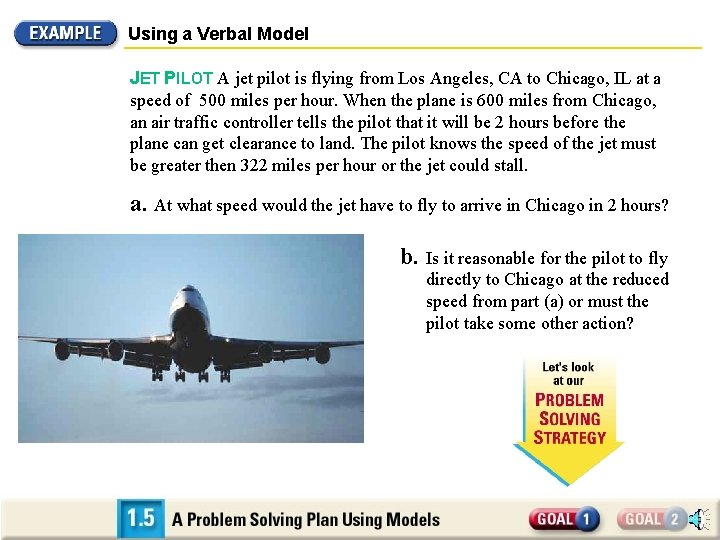# TRANSLATING VERBAL PHRASES Verbal Phrase Expression The sum

• Slides: 10TRANSLATING VERBAL PHRASES Verbal Phrase Expression The sum of six and a number 6+x Eight more than a number y+8 A number plus five n+5 A number increased by seven x+7 A number decreased by nine n– 9 Ten times a number Seven divided by a number 10 • n or (10 n) (10 (10 n 7 xUSING A VERBAL MODEL In Mathematics there is a difference between a phrase and a sentence. Phrases translate into expressions; sentences translate into equations or inequalities. Phrases Expressions Sentences Equations or InequalitiesUSING A VERBAL MODEL Phrase Expression The sum of six and a number is Sentence 6+x= In this sentence, “is” says that one quantity is equal to one another. Equation The sum of six and a number is is twelve. Sentence In this sentence, the words “is less than” indicate an inequality. The sum of six and a number is less thantwelve. 6 + x = 12 Inequality 6 + x < 12USING A VERBAL MODEL Writing algebraic expressions, equations, or inequalities that represent real-life situations is called modeling. The expression, equation, or inequality is a mathematical model. Use three steps to write a mathematical model. WRITE A ASSIGN WRITE AN VERBAL MODEL. LABELS. ALGEBRAIC MODEL.Writing an Algebraic Model You and three friends are having a dim sum lunch at a Chinese restaurant that charges \$2 per plate. You order lots of plates. The waiter gives you a bill for \$25. 20, which includes tax of \$1. 20. Use mental math to solve the equation for how many plates your group ordered. SOLUTION Understand the problem situation before you begin. For example, notice that tax is added after the total cost of the dim sum plates is figured.Writing an Algebraic Model VERBAL MODEL Cost per plate LABELS Cost per plate = 2 (dollars) Number of plates = p (plates) • Number of plates = Bill – Tax Amount of bill = 25. 20 (dollars) Tax = 1. 20 ALGEBRAIC MODEL 2 p = 25. 20 (dollars) – 1. 20 2 p = 24. 00 p = 12 Your group ordered 12 plates of food costing \$24. 00.Writing an Algebraic Model A PROBLEM SOLVING PLAN USING MODELS VERBAL MODEL Ask yourself what you need to know to solve the problem. Then write a verbal model that will give you what you need to know. LABELS Assign labels to each part of your verbal problem. ALGEBRAIC Use the labels to write an algebraic model based on MODEL your verbal model. SOLVE Solve the algebraic model and answer the original question. CHECK Check that your answer is reasonable.Using a Verbal Model JET PILOT A jet pilot is flying from Los Angeles, CA to Chicago, IL at a speed of 500 miles per hour. When the plane is 600 miles from Chicago, an air traffic controller tells the pilot that it will be 2 hours before the plane can get clearance to land. The pilot knows the speed of the jet must be greater then 322 miles per hour or the jet could stall. a. At what speed would the jet have to fly to arrive in Chicago in 2 hours? b. Is it reasonable for the pilot to fly directly to Chicago at the reduced speed from part (a) or must the pilot take some other action?Using a Verbal Model a. At what speed would the jet have to fly to arrive in Chicago in 2 hours? SOLUTION You can use the formula (rate)(time) = (distance) to write a verbal model. VERBAL MODEL Speed of jet LABELS Speed of jet = x (miles per hour) Time = 2 (hours) Distance to travel = 600 (miles) ALGEBRAIC MODEL • Time = Distance to travel 2 x = 600 x = 300 To arrive in 2 hours, the pilot would have to slow the jet down to 300 miles per hour.Using a Verbal Model b. Is it reasonable for the pilot to fly directly to Chicago at 300 miles per hour or must the pilot take some other action? It is not reasonable for the pilot to fly at 300 miles per hour, because the jet could stall. The pilot should take some other action, such as circling in a holding pattern, to use some of the time.